## 8.1 Capital Budgeting and Decision Making

### Learning Objective

1. Apply the concept of the time value of money to capital budgeting decisions.

Question: What is the difference between management decisions made in Chapter 7 "How Are Relevant Revenues and Costs Used to Make Decisions?" and management decisions made in this chapter?

Answer: The types of decisions covered in this chapter and Chapter 7 "How Are Relevant Revenues and Costs Used to Make Decisions?" are similar in that they require an analysis of differential revenues and costs. However, Chapter 7 "How Are Relevant Revenues and Costs Used to Make Decisions?" involves short-run operating decisions (e.g., special orders from customers), while this chapter focuses on long-run capacity decisions (e.g., purchasing long-lived assets to increase capacity for many years).

Organizations make a variety of long-run investment decisions. The San Francisco Symphony invests in stage risers for its orchestra members. McDonald’s invests in new restaurants. Honda Motor Co. invests in new manufacturing facilities. Bank of America invests in new branches. These examples have one common feature: all of these companies are investing in assets that will affect the organization for several years.

Question: The process of analyzing and deciding which long-term investments to make is called a capital budgeting decisionThe process of analyzing and deciding which long-term investments (or capital expenditure decision) to make., also known as a capital expenditure decision. Capital budgeting decisions involve using company funds (capital) to invest in long-term assets. How does the evaluation of these types of capital budgeting decisions differ from short-term operating decisions discussed in Chapter 7 "How Are Relevant Revenues and Costs Used to Make Decisions?"?

Answer: When looking at capital budgeting decisions that affect future years, we must consider the time value of money. The time value of money concept is the premise that a dollar received today is worth more than a dollar received in the future. To clarify this point, suppose a friend owes you $100. Would you prefer to receive$100 today or 3 years from today? The money is worth more to you if you receive it today because you can invest the $100 for 3 years. For capital budgeting decisions, the issue is how to value future cash flows in today’s dollars. The term cash flowThe amount of cash received or paid at a specific point in time. refers to the amount of cash received or paid at a specific point in time. The term present valueThe term used to describe future cash flows (both in and out) in today’s dollars. describes the value of future cash flows (both in and out) in today’s dollars. ### Business in Action 8.1 Capital Budgeting Decisions at JCPenney and Kohl’s JCPenney Company has over 1,000 department stores in the United States, and Kohl’s Corporation has over 800. Both companies cater to a “middle market.” In October 2006, Kohl’s announced plans to open 65 new stores. At about the same time, JCPenney announced plans to open 20 new stores, 17 of which would be stand-alone stores. This was a departure from JCPenney’s typical approach of serving as an anchor store for regional shopping malls. The decision to open new stores is an example of a capital budgeting decision because management must analyze the cash flows associated with the new stores over the long term. When managers evaluate investments in long-term assets, they want to know how much cash would be spent on the investment and how much cash would be received as a result of the investment. The investment proposal is likely rejected if cash inflows do not exceed cash outflows. (Think about a personal investment. If you would receive only$700 in the future from an investment of $1,000 today, you undoubtedly would not make the investment because you would lose$300!) If cash inflows are expected to exceed cash outflows, managers must consider when the cash inflows and outflows occur before taking on the investment. (Again, consider an investment of $1,000 today. If you expect to receive$1,050 in 20 years rather than at the end of 1 year, you would probably think twice before investing because it would take 20 years to make $50!) Question: We use two methods to evaluate long-term investments, both of which consider the time value of money. What are these two methods? Answer: The first is called the net present value (NPV) method, and the second is called the internal rate of return method. Before presenting these two methods, let’s discuss the time value of money (present value) concepts. ## The Present Value Formula Question: Suppose you invest$1,000 for 1 year at an interest rate of 5 percent per year, as shown in the following timeline. How much will you have at the end of 1 year (or what is the future value of the investment)?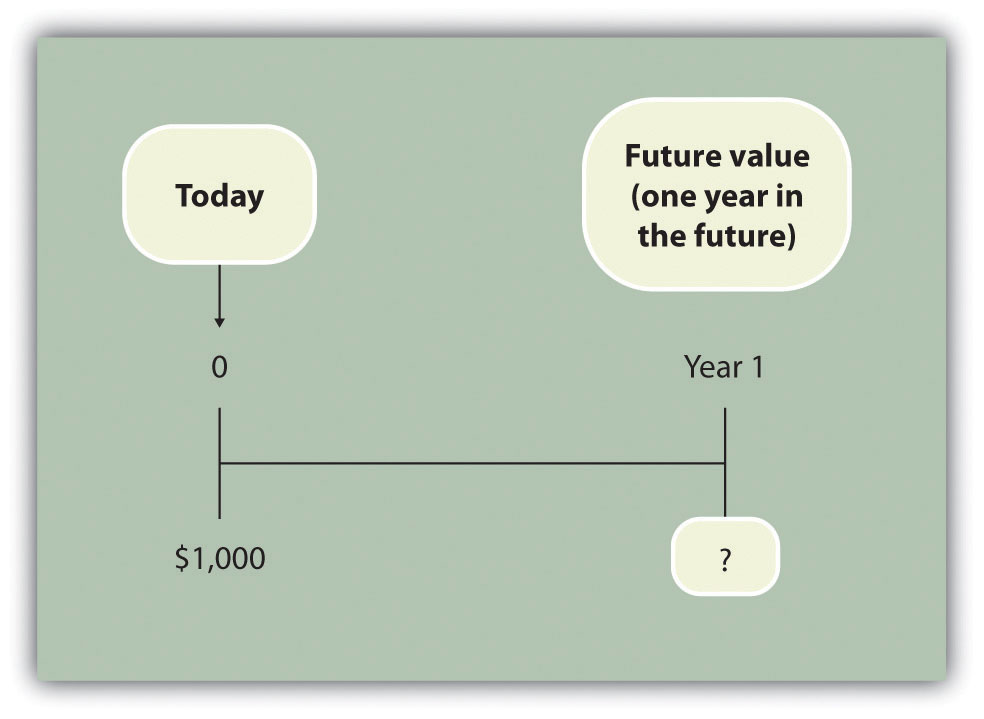Answer: You will have $1,050:$1,050 = $1,000 × (1 + .05) Question: Let’s change course and find the present value of the same future cash flow. If you receive$1,050 in 1 year, how much is that worth in today’s dollars assuming an annual interest rate of 5 percent?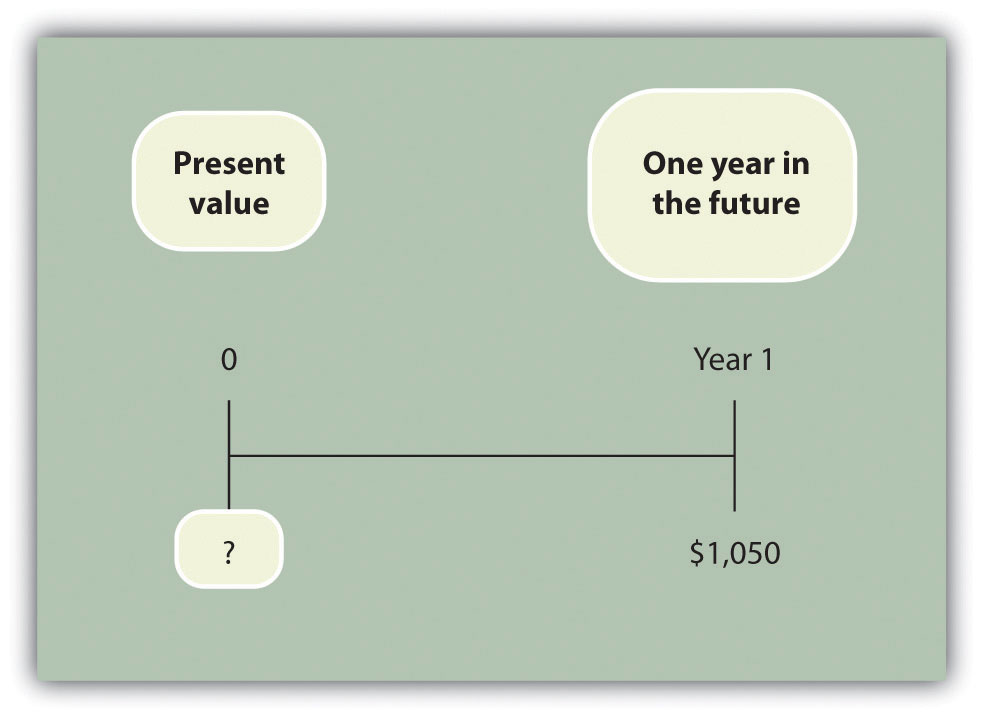Answer: The present value is $1,000, calculated as follows: $1,000=1,050(1+.05)$ Question: Let’s go back to finding a future value. Assume you invest$1,000 today at an annual rate of 5 percent for 2 years. How much will you have at the end of 2 years?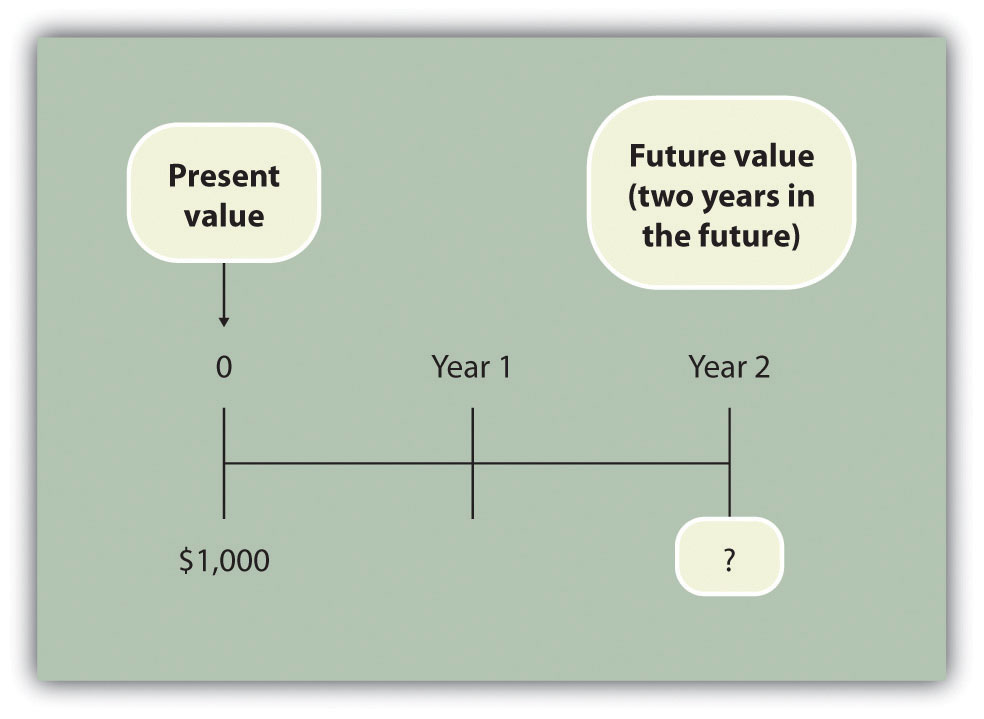Answer: At the end of 1 year, you will have $1,050 (=$1,000 × [1 + .05]). At the end of the second year, you will have $1,102.50, which is$1,050 × (1 + .05). The equation is

$1,102.50 =$1,000 × (1 + .05) × (1 + .05) or $1,102.50 =$1,000 × (1 + .05)2

Question: Again, let’s change course and find the present value of the same future cash flow. If you receive $1,102.50 in 2 years, how much is that worth in today’s dollars assuming an annual interest rate of 5 percent?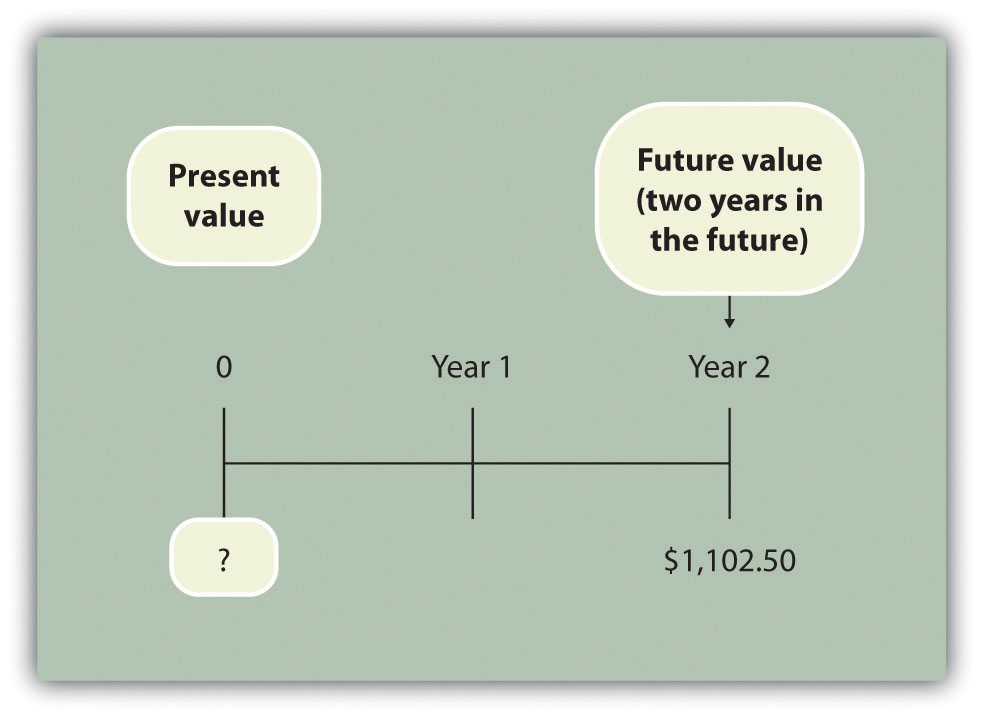Answer: The present value is$1,000, calculated as follows:

$1,000=1,102.50(1+.05)2$

These examples show that one equation can be used to find the present value of a future cash flow. The equation is

### Key Equation

$P=Fn(1+r)n$

where

P = Present value of an amount Fn = Amount received n years in the future r = Annual interest rate n = Number of years

Question: Let’s use this formula to solve for the following: Assume $500 will be received 4 years from today, and the annual interest rate is 10 percent. What is the present value of this cash flow?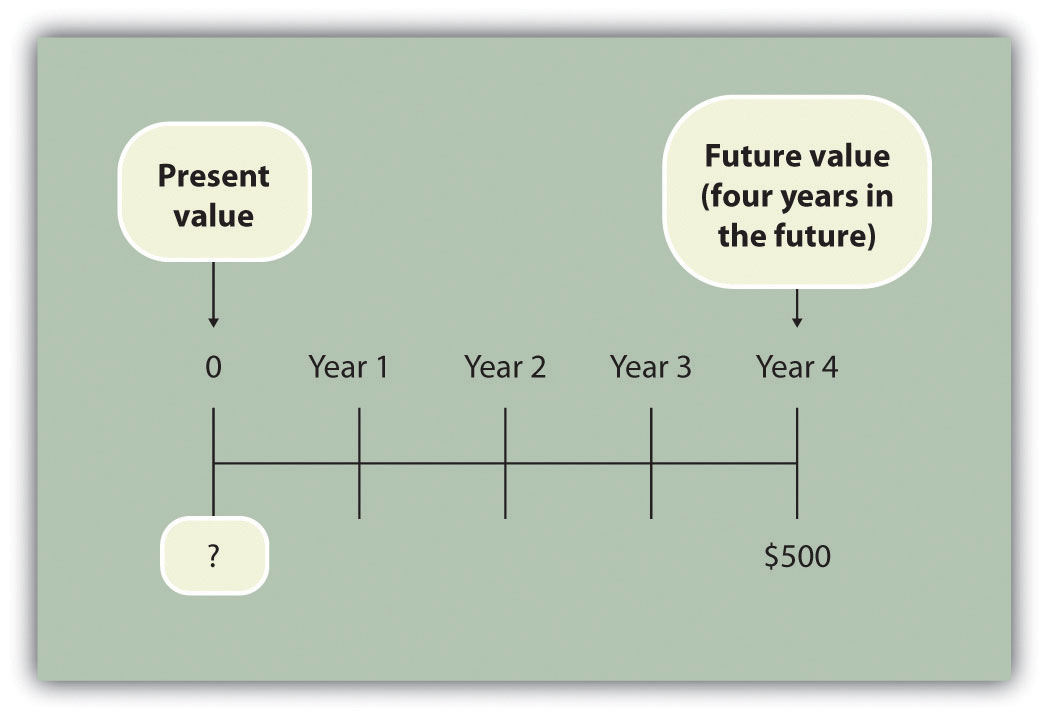Answer: The present value is$341.51, calculated as follows:

$P=Fn(1+r)n=500(1+.10)4=5001.4641=341.51$

## Present Value Tables

Question: Although most managers use spreadsheets, such as Excel, to perform present value calculations (discussed later in this chapter), you can also use the present value tables in the appendix to this chapter, labeled Figure 8.9 "Present Value of $1 Received at the End of " and Figure 8.10 "Present Value of a$1 Annuity Received at the End of Each Period for ", for these calculations. Figure 8.9 "Present Value of $1 Received at the End of " simply provides the present value of$1 (i.e., F = $1) given the number of years (n) and the interest rate (r). How are these tables used to calculate present value amounts? Answer: Let’s look at an example to see how these tables work. Assume$1 will be received 4 years from today (n = 4), and the interest rate is 10 percent (r = 10 percent). What is the present value of this cash flow? Look at Figure 8.9 "Present Value of $1 Received at the End of " in the appendix. Find the column labeled 10 percent and the row labeled 4. The present value is$0.6830, or $0.68 rounded. The table amount given is often called a factor. The factor in this example is 0.6830 (note that the formula to find this factor is shown at the top of Figure 8.9 "Present Value of$1 Received at the End of ").

Now assume all the same facts, except that $500 rather than$1 will be received in 4 years. To find the present value, simply multiply the factor found in Figure 8.9 "Present Value of $1 Received at the End of " by$500, as follows:

Notice that this present value is the same as the one we calculated using the formula P = Fn ÷ (1 + r)n, with the exception of a small difference due to rounding the factor in Figure 8.9 "Present Value of $1 Received at the End of ". Next, we use present value concepts to evaluate projects with the NPV method. ### Key Takeaway • Present value calculations tell us the value of future cash flows in today’s dollars. The present value of a cash flow can be calculated by using the formula P = Fn ÷ (1 + r)n. It can also be calculated by using the tables in the appendix of this chapter. Simply find the factor in Figure 8.9 "Present Value of$1 Received at the End of " given the number of years (n) and annual interest rate (r). Then multiply the factor by the future cash flow, as follows:

Present value = Amount received in the future × Present value factor

### Review Problem 8.1

For each of the following independent scenarios, calculate the present value of the cash flow described. Round to the nearest dollar.

1. You will receive $5,000, 5 years from today, and the interest rate is 8 percent. 2. You will receive$80,000, 9 years from today, and the interest rate is 10 percent.
3. You will receive $400,000, 20 years from today, and the interest rate is 20 percent. 4. You will receive$250,000, 10 years from today, and the interest rate is 15 percent.

Solution to Review Problem 8.1

Two approaches can be used to find the present value of a cash flow. The first requires using the formula P = Fn ÷ (1 + r)n. The second requires using Figure 8.9 "Present Value of $1 Received at the End of " in the appendix to find the present value factor and inserting it in the following formula: Present value = Amount received in the future × Present value factor (from Figure 8.9 "Present Value of$1 Received at the End of ")

We show both approaches in the following solutions.

1. Using the formula P = Fn ÷ (1 + r)n, we get

$3,403=5,000÷(1+.08)5$
2. Using the formula P = Fn ÷ (1 + r)n, we get

$33,928=80,000÷(1+.10)9$
3. The small difference between the two approaches is due to rounding the factor in Figure 8.9 "Present Value of $1 Received at the End of ". Using the formula P = Fn ÷ (1 + r)n, we get $10,434=400,000÷(1+.20)20$ 4. The small difference between the two approaches is due to rounding the factor Figure 8.9 "Present Value of$1 Received at the End of ".

Using the formula P = Fn ÷ (1 + r)n, we get

$61,796=250,000÷(1+.15)10$